# Chaos

deterministic chaos.

Chaos describes a situation where typical solutions (or orbits) of a differential equation (or typical evolutions of some other model describing deterministic evolution) do not converge to a stationary or periodic function (of time) but continue to exhibit a seemingly unpredictable behaviour. There are different ways to formalize this notion mathematically. Below one such a formalization will be considered, followed by a discussion of some examples.

The dynamical systems (or models describing deterministic evolution, cf. Dynamical system) considered are differential equations, with,a differentiable manifold and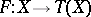a vector field on, and differentiable mappings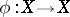which may or may not be invertible. For a given initial state, the corresponding evolution is the solution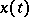of the differential equation with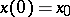or, in the case of a mapping, the function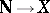given by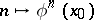. The last case is the discrete-time situation, the first case that of continuous time. Even if the evolutions can be defined for negative time, only the part with positive time is considered. Also, only bounded evolutions are considered here, i.e. evolutions,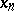, with, respectively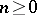, whose closure, as a subset of, is compact. It is assumed that there is a metric defined on.

One says that such a dynamical system is chaotic if there is a subset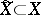which has positive measure (for every measure in the Lebesgue measure class) which is invariant in the sense that every evolution starting in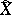stays in, and such that the evolutions inhave the following properties:

1) no evolution starting inis periodic or quasi-periodic; an evolutionis quasi-periodic if it can be written as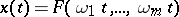with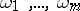independent over the rationals and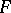periodic with period 1 in all its variables, an evolutionis quasi-periodic if it can be written as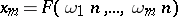with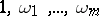independent over the rationals andperiodic with period 1 in all its variables;

2) no evolution intends to a periodic or quasi-periodic evolution as time tends to infinity;

3) (sensitive dependence on initial conditions) there is some positive constantsuch that for eachand each, there is some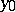, in an-neighbourhood of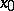, such that for some positive time the evolutions starting inandare more thanapart.

These conditions are probably not independent: the first two conditions may be a consequence of the third condition, but this has not been proved (1988). The third condition implies some inpredictability. Even if one knows the initial state with arbitrary (but finite) precision, there is some moment in the future at which the state cannot be predicted within a distancefrom the information about the initial state.

General references for this area are [a1] and [a4].

The main examples of chaotic dynamical systems (and dynamical systems which are supposed to be chaotic) are discussed below.

1) The logistic family. This is a one-parameter family of one-dimensional mappings: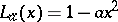. It has been proved that for a large set (of positive Lebesgue measure) of values of the parameter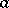, this mapping defines a chaotic dynamical system. These mappings were introduced to describe population dynamics under certain conditions. A general reference is [a3].

2) The Hénon family [a5]. This is a two-parameter family of two-dimensional invertible mappings,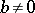. In this example there is only numerical evidence that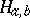defines, for many parameter valuesand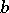, a chaotic dynamical system. The mathematical analysis of this example is still in progress (1988).

3) The Lorenz family [a6]. This is a three-parameter family of differential equations in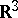: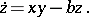There is a well-developed theory concerning this system. Still, there is no complete proof that for any of the parameter values this system is chaotic. This chaoticity is strongly suggested by numerical results combined with geometric arguments: what is lacking is a tedious numerical verification. This equation was proposed in connection with convection problems.

4) General (non-trivial) Axiomattractors. This is a class of abstract dynamical systems which are chaotic. Among the chaotic dynamical systems they are the most "regular" and also they are the ones which are mathematically well understood, see [a2].

Finally, in a number of physical and chemical experiments, in particular related with weak turbulence and open chemical reactions far from equilibrium, the experimental data indicate that one should explain these experiments in terms of chaotic dynamical systems, see [a1].

The literature has not yet standardized with respect to the definition of chaotic mappings. Thus, in [a7] the discrete-time system ongiven by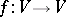is said to be chaotic if (i)has sensitive dependence on initial conditions; (ii)is topologically transitive, i.e. for every open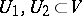there is an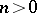such that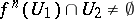; and (iii) the periodic points are dense in.

There is a second notion, principally in physics and probability, which also goes by the name chaos; cf. Wiener chaos decomposition.